# 3 DIFFERENTIATION RULES DIFFERENTIATION RULES We have Seen

• Slides: 78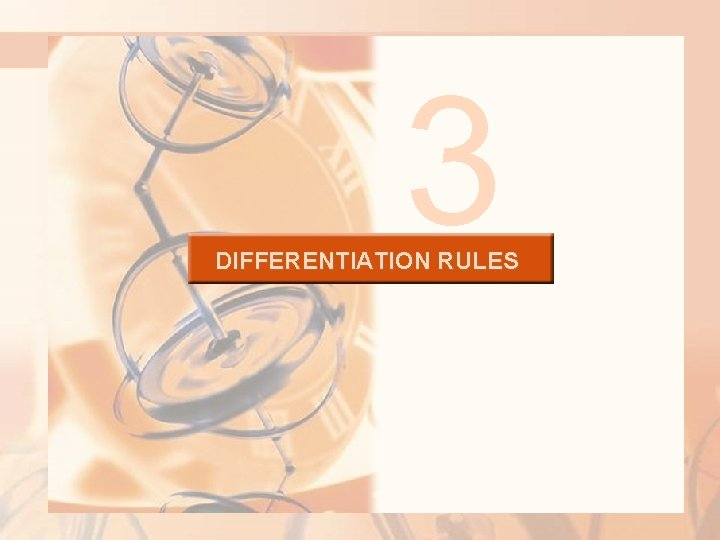3 DIFFERENTIATION RULESDIFFERENTIATION RULES We have: § Seen how to interpret derivatives as slopes and rates of change § Seen how to estimate derivatives of functions given by tables of values § Learned how to graph derivatives of functions that are defined graphically § Used the definition of a derivative to calculate the derivatives of functions defined by formulas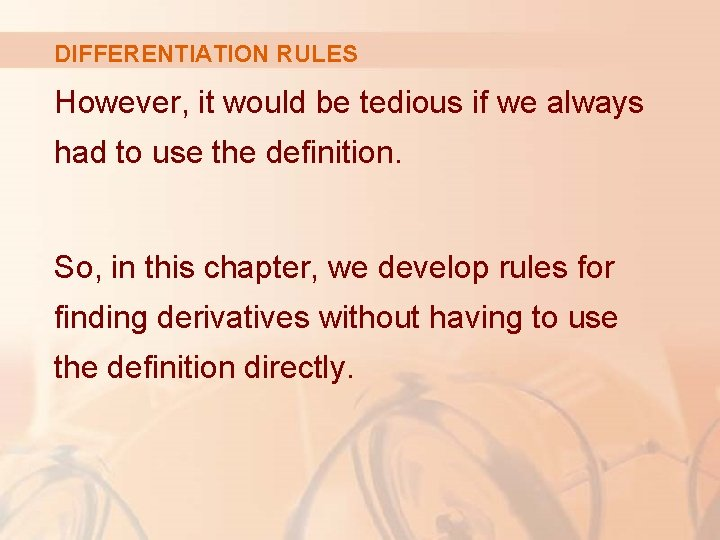DIFFERENTIATION RULES However, it would be tedious if we always had to use the definition. So, in this chapter, we develop rules for finding derivatives without having to use the definition directly.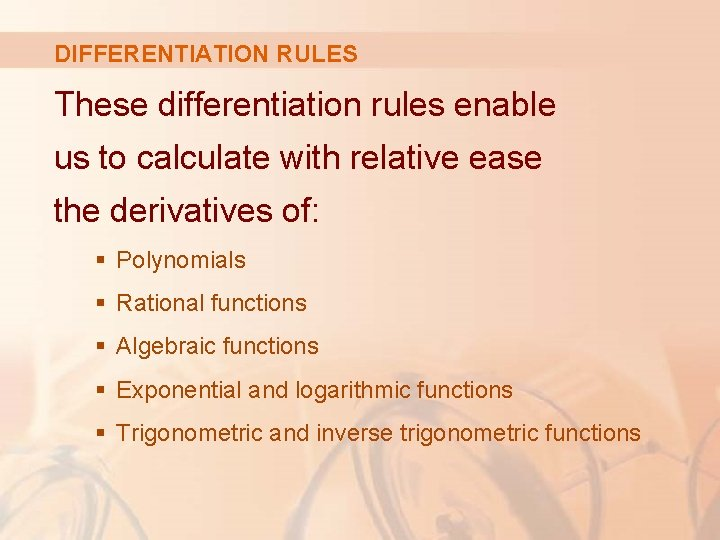DIFFERENTIATION RULES These differentiation rules enable us to calculate with relative ease the derivatives of: § Polynomials § Rational functions § Algebraic functions § Exponential and logarithmic functions § Trigonometric and inverse trigonometric functionsDIFFERENTIATION RULES We then use these rules to solve problems involving rates of change and the approximation of functions.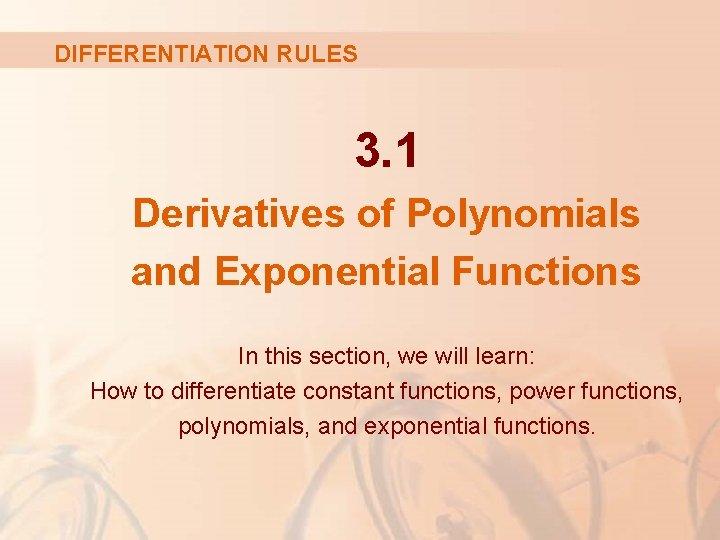DIFFERENTIATION RULES 3. 1 Derivatives of Polynomials and Exponential Functions In this section, we will learn: How to differentiate constant functions, power functions, polynomials, and exponential functions.CONSTANT FUNCTION Let’s start with the simplest of all functions—the constant function f(x) = c.CONSTANT FUNCTION The graph of this function is the horizontal line y = c, which has slope 0. § So, we must have f’(x) = 0.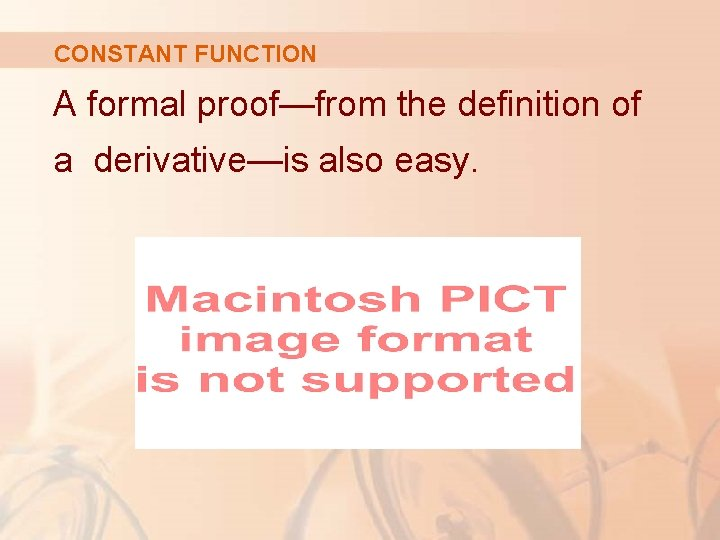CONSTANT FUNCTION A formal proof—from the definition of a derivative—is also easy.DERIVATIVE In Leibniz notation, we write this rule as follows.POWER FUNCTIONS We next look at the functions f(x) = xn, where n is a positive integer.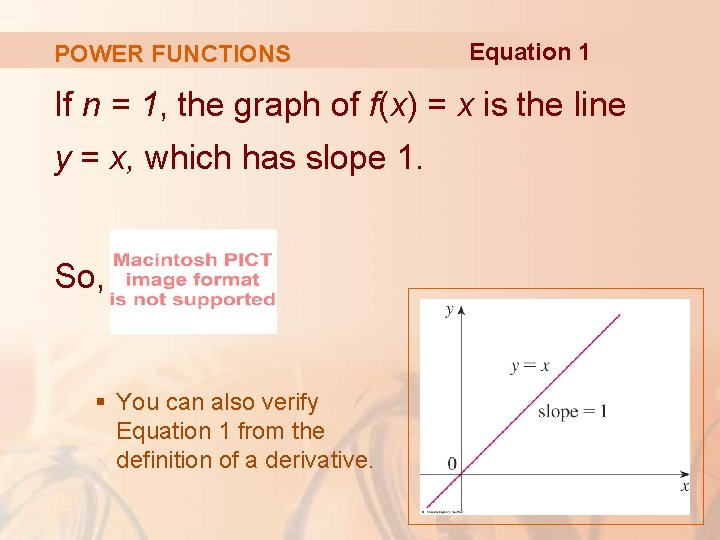POWER FUNCTIONS Equation 1 If n = 1, the graph of f(x) = x is the line y = x, which has slope 1. So, § You can also verify Equation 1 from the definition of a derivative.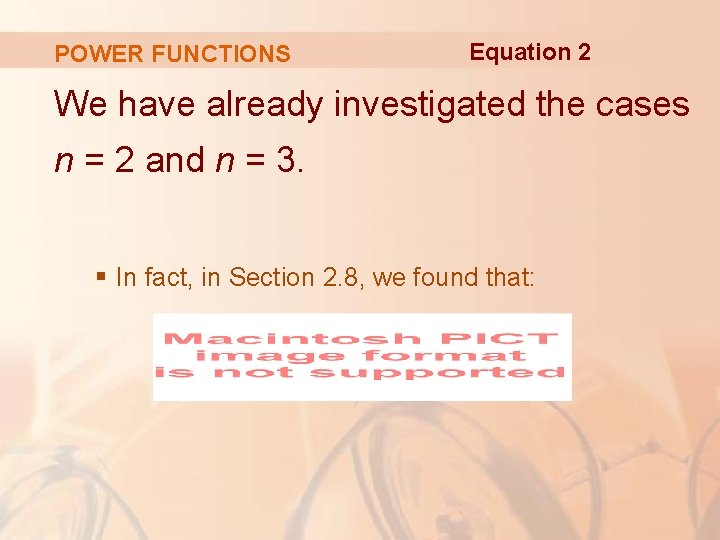POWER FUNCTIONS Equation 2 We have already investigated the cases n = 2 and n = 3. § In fact, in Section 2. 8, we found that: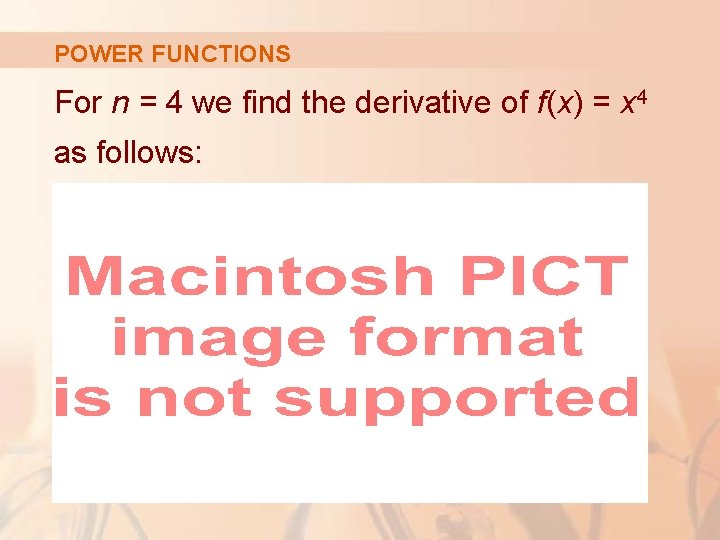POWER FUNCTIONS For n = 4 we find the derivative of f(x) = x 4 as follows:POWER FUNCTIONS Thus, Equation 3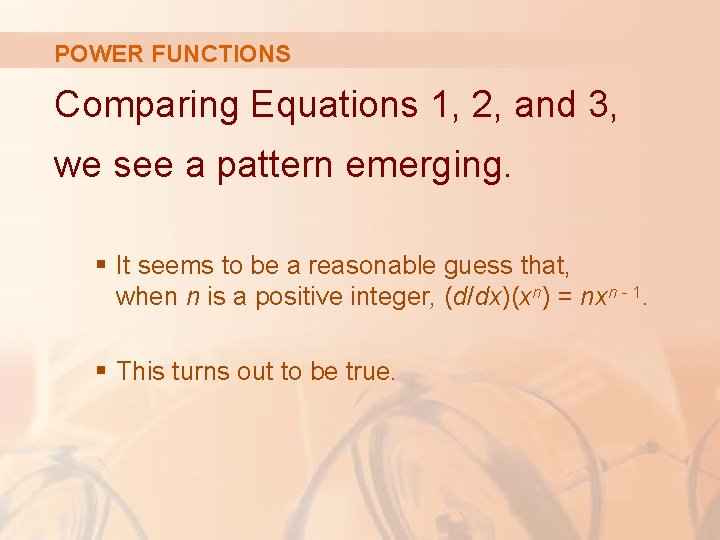POWER FUNCTIONS Comparing Equations 1, 2, and 3, we see a pattern emerging. § It seems to be a reasonable guess that, when n is a positive integer, (d/dx)(xn) = nxn - 1. § This turns out to be true.POWER RULE If n is a positive integer, then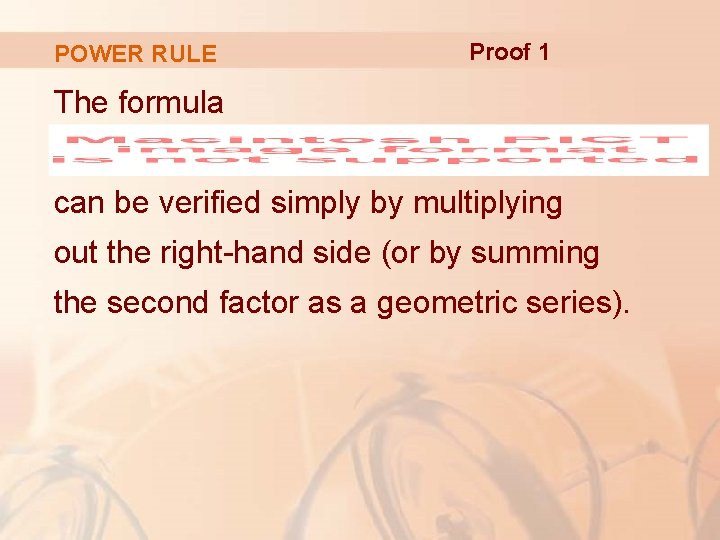POWER RULE Proof 1 The formula can be verified simply by multiplying out the right-hand side (or by summing the second factor as a geometric series).POWER RULE Proof 1 If f(x) = xn, we can use Equation 5 in Section 2. 7 for f’(a) and the previous equation to write: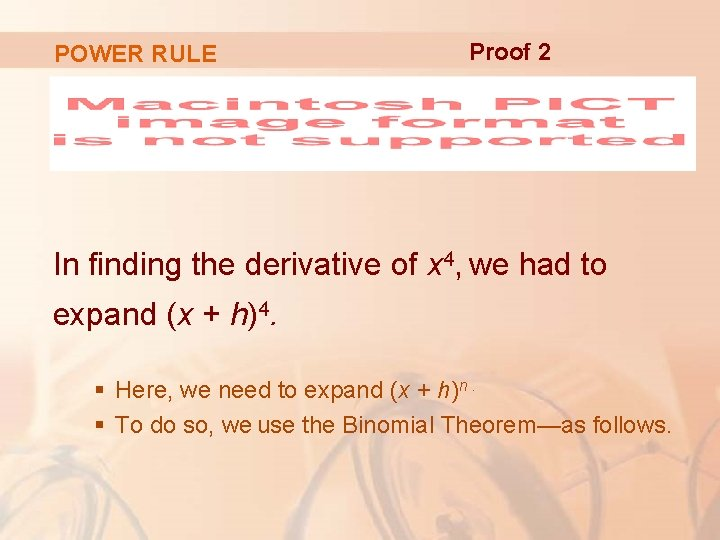POWER RULE Proof 2 In finding the derivative of x 4, we had to expand (x + h)4. § Here, we need to expand (x + h)n. § To do so, we use the Binomial Theorem—as follows.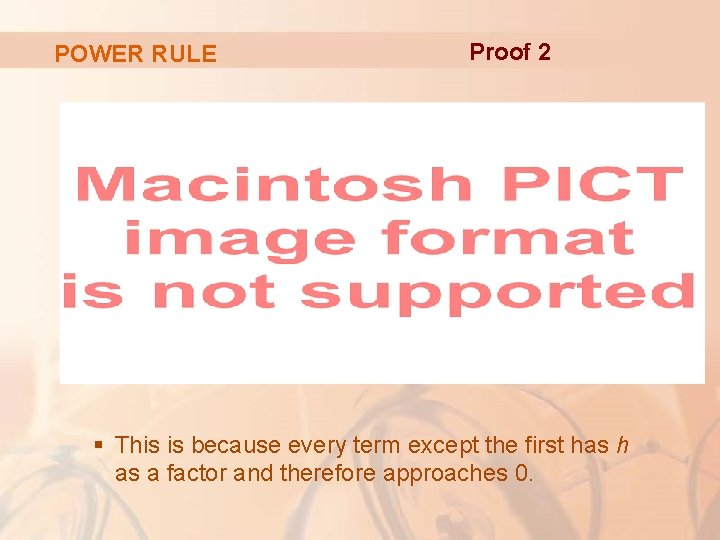POWER RULE Proof 2 § This is because every term except the first has h as a factor and therefore approaches 0.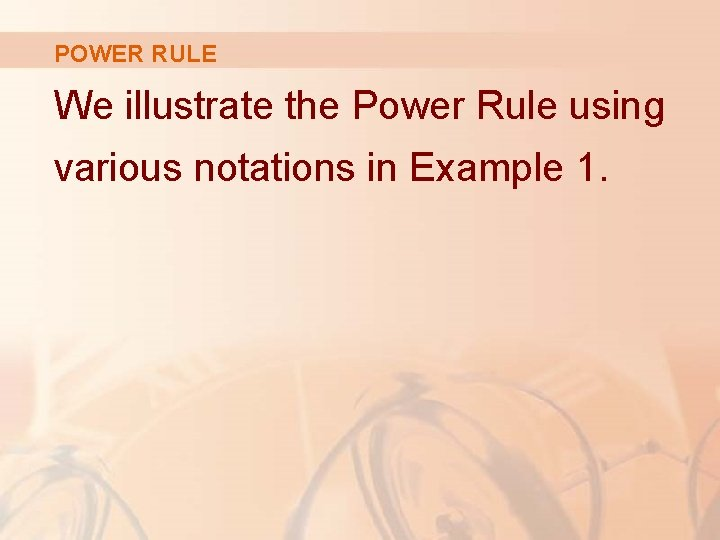POWER RULE We illustrate the Power Rule using various notations in Example 1.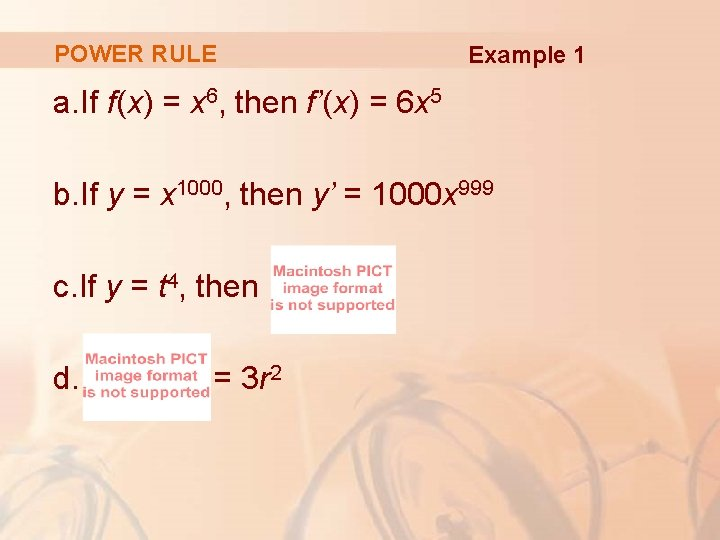POWER RULE Example 1 a. If f(x) = x 6, then f’(x) = 6 x 5 b. If y = x 1000, then y’ = 1000 x 999 c. If y = t 4, then d. = 3 r 2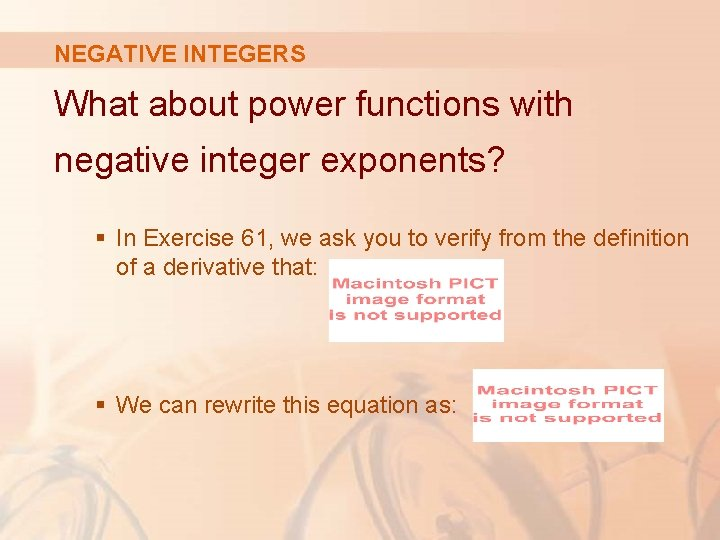NEGATIVE INTEGERS What about power functions with negative integer exponents? § In Exercise 61, we ask you to verify from the definition of a derivative that: § We can rewrite this equation as:NEGATIVE INTEGERS So, the Power Rule is true when n = -1. § In fact, we will show in the next section that it holds for all negative integers.FRACTIONS What if the exponent is a fraction? § In Example 3 in Section 2. 8, we found that: § This can be written as: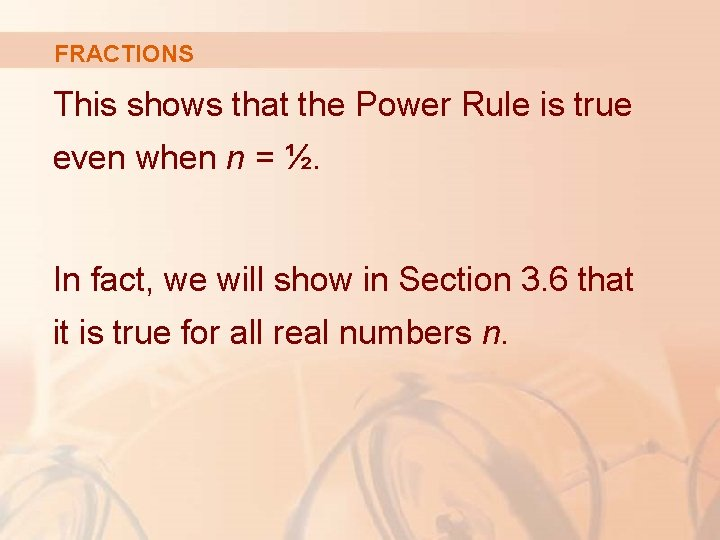FRACTIONS This shows that the Power Rule is true even when n = ½. In fact, we will show in Section 3. 6 that it is true for all real numbers n.POWER RULE—GENERAL VERSION If n is any real number, then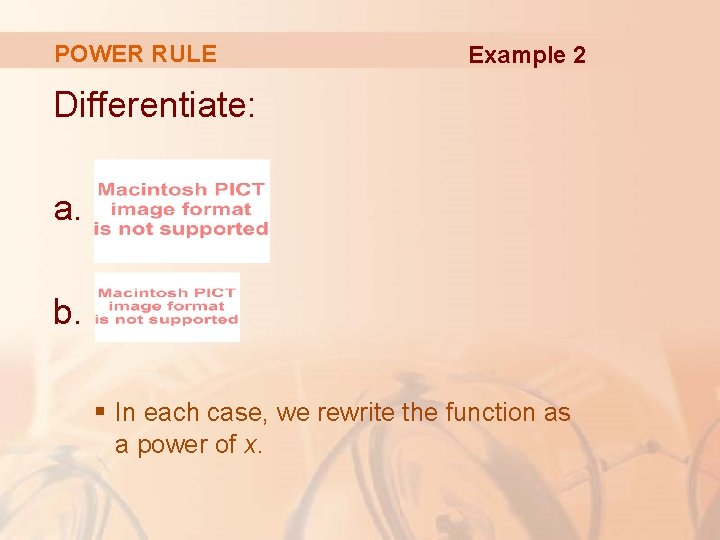POWER RULE Example 2 Differentiate: a. b. § In each case, we rewrite the function as a power of x.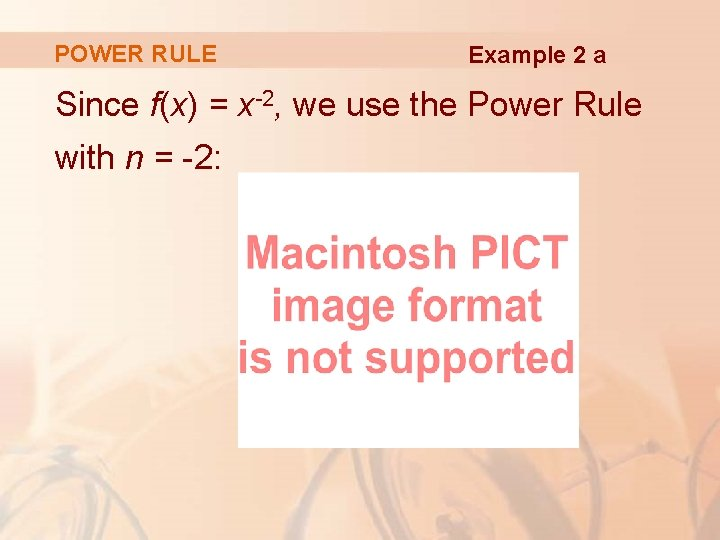POWER RULE Example 2 a Since f(x) = x-2, we use the Power Rule with n = -2:POWER RULE Example 2 b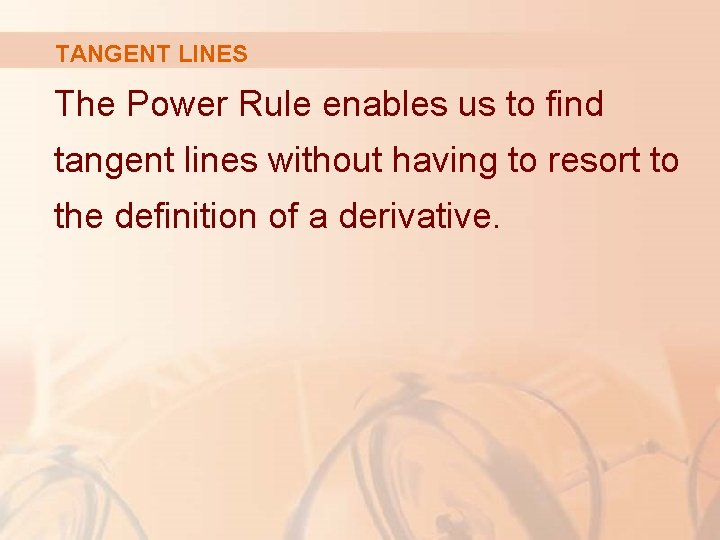TANGENT LINES The Power Rule enables us to find tangent lines without having to resort to the definition of a derivative.NORMAL LINES It also enables us to find normal lines. § The normal line to a curve C at a point P is the line through P that is perpendicular to the tangent line at P. § In the study of optics, one needs to consider the angle between a light ray and the normal line to a lens.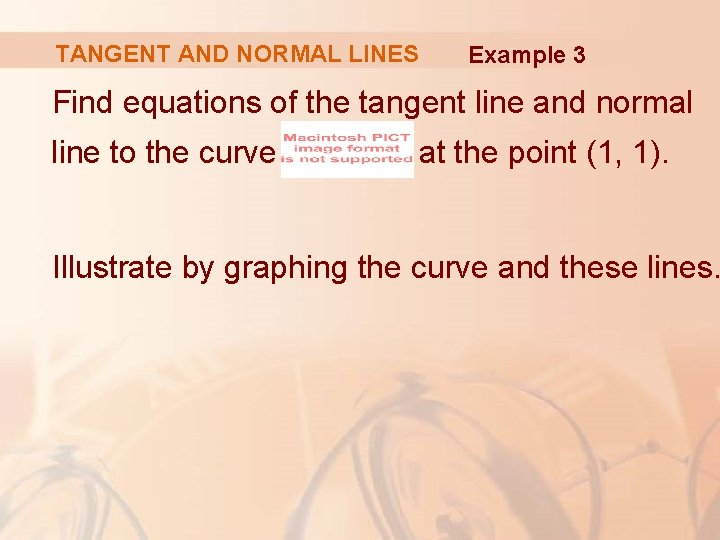TANGENT AND NORMAL LINES Example 3 Find equations of the tangent line and normal line to the curve at the point (1, 1). Illustrate by graphing the curve and these lines.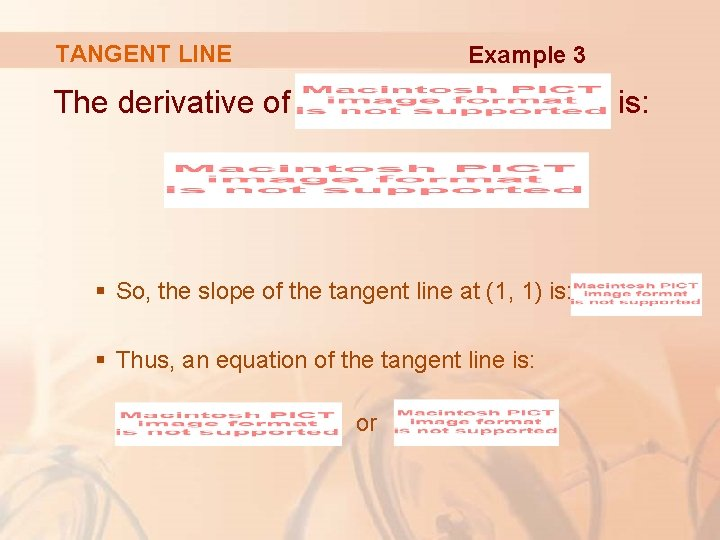TANGENT LINE Example 3 The derivative of is: § So, the slope of the tangent line at (1, 1) is: § Thus, an equation of the tangent line is: or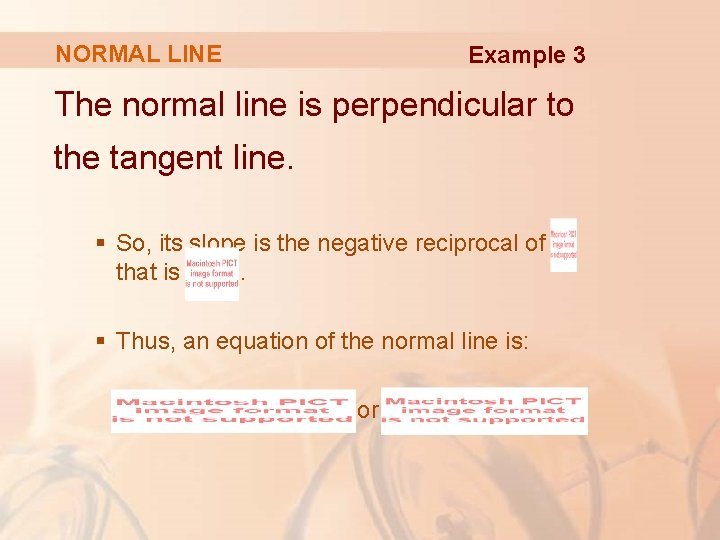NORMAL LINE Example 3 The normal line is perpendicular to the tangent line. § So, its slope is the negative reciprocal of that is. § Thus, an equation of the normal line is: or ,TANGENT AND NORMAL LINES Example 3 We graph the curve and its tangent line and normal line here.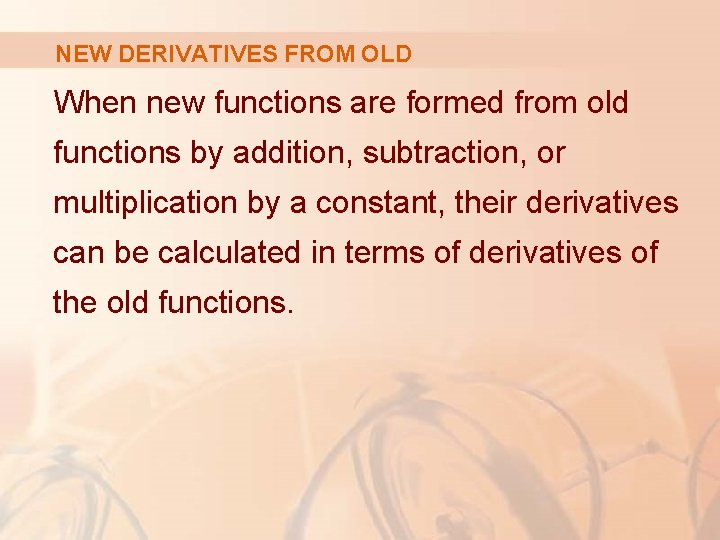NEW DERIVATIVES FROM OLD When new functions are formed from old functions by addition, subtraction, or multiplication by a constant, their derivatives can be calculated in terms of derivatives of the old functions.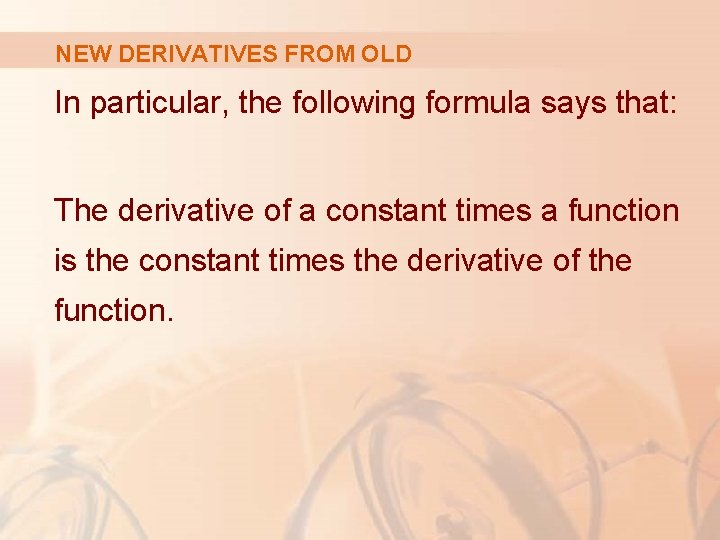NEW DERIVATIVES FROM OLD In particular, the following formula says that: The derivative of a constant times a function is the constant times the derivative of the function.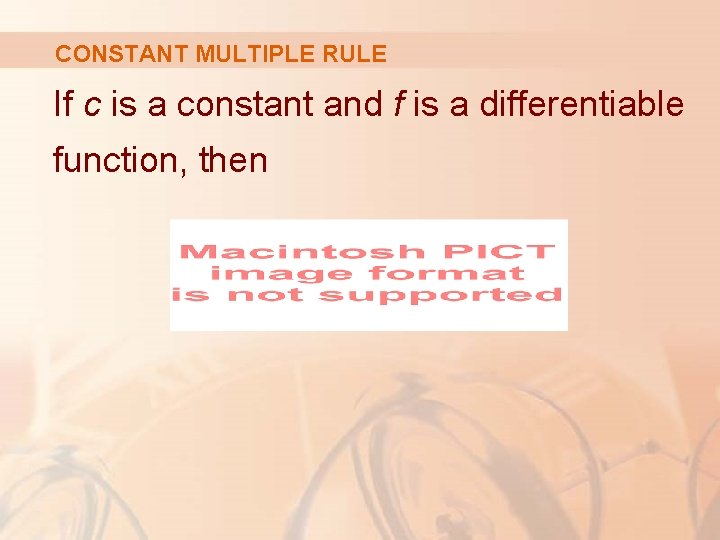CONSTANT MULTIPLE RULE If c is a constant and f is a differentiable function, thenCONSTANT MULTIPLE RULE Let g(x) = cf(x). Then, ProofNEW DERIVATIVES FROM OLD Example 4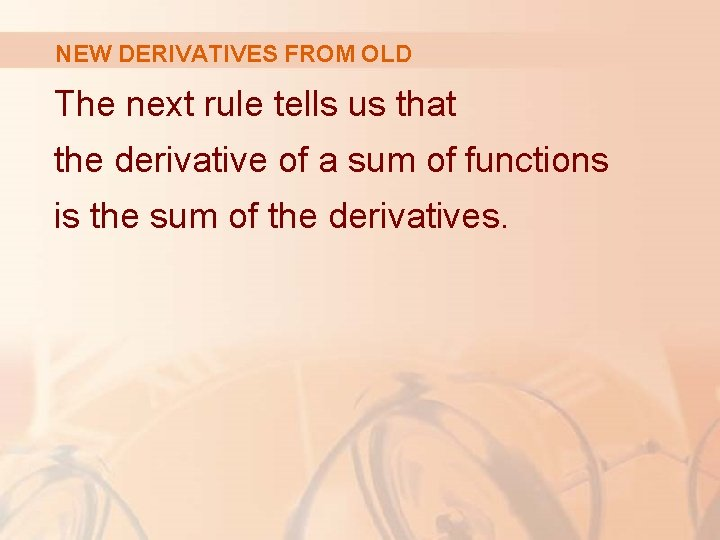NEW DERIVATIVES FROM OLD The next rule tells us that the derivative of a sum of functions is the sum of the derivatives.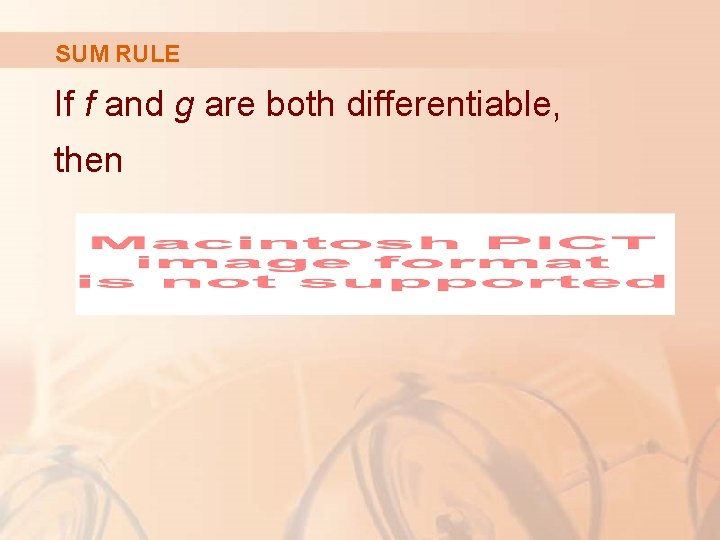SUM RULE If f and g are both differentiable, then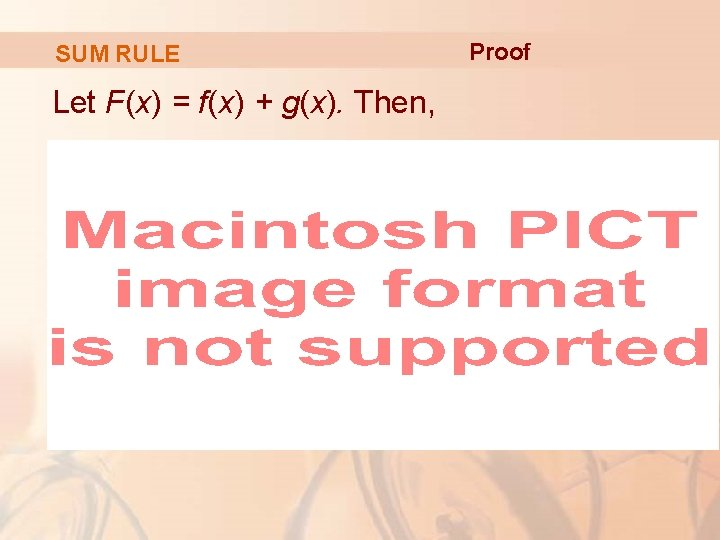SUM RULE Let F(x) = f(x) + g(x). Then, ProofSUM RULE The Sum Rule can be extended to the sum of any number of functions. § For instance, using this theorem twice, we get: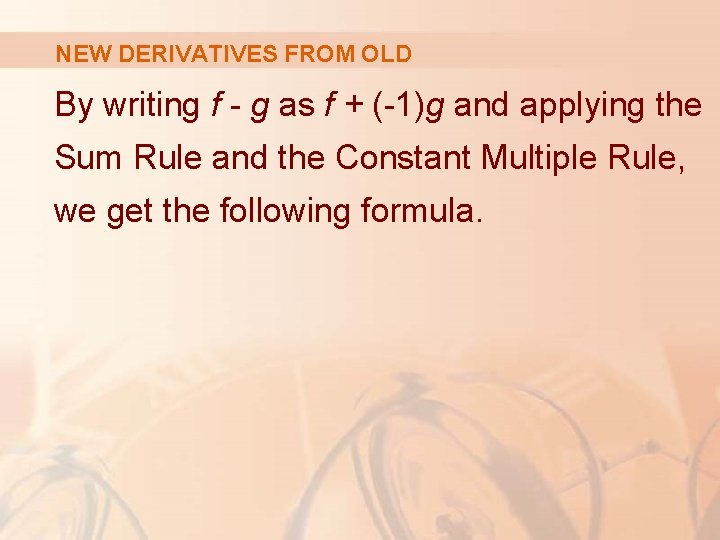NEW DERIVATIVES FROM OLD By writing f - g as f + (-1)g and applying the Sum Rule and the Constant Multiple Rule, we get the following formula.DIFFERENCE RULE If f and g are both differentiable, then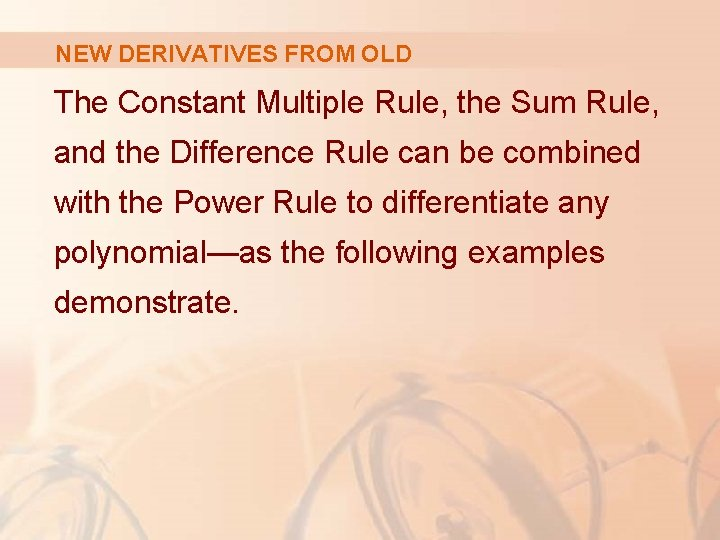NEW DERIVATIVES FROM OLD The Constant Multiple Rule, the Sum Rule, and the Difference Rule can be combined with the Power Rule to differentiate any polynomial—as the following examples demonstrate.NEW DERIVATIVES FROM OLD Example 5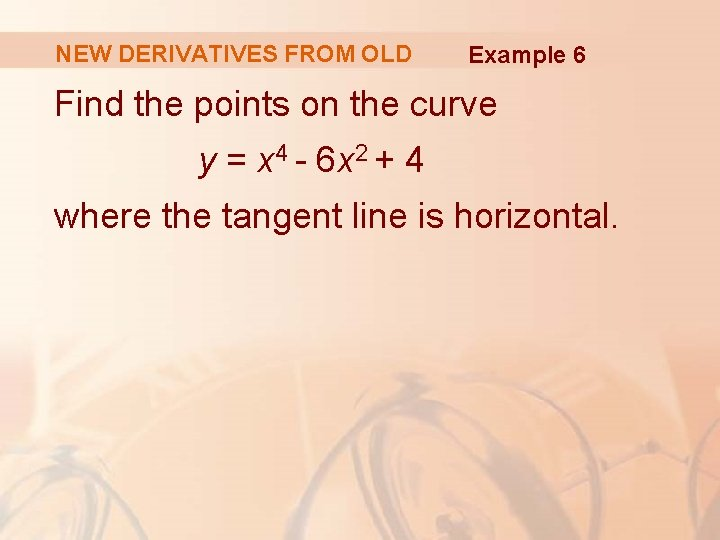NEW DERIVATIVES FROM OLD Example 6 Find the points on the curve y = x 4 - 6 x 2 + 4 where the tangent line is horizontal.NEW DERIVATIVES FROM OLD Example 6 Horizontal tangents occur where the derivative is zero. § We have: § Thus, dy/dx = 0 if x = 0 or x 2 – 3 = 0, that is, x = ± .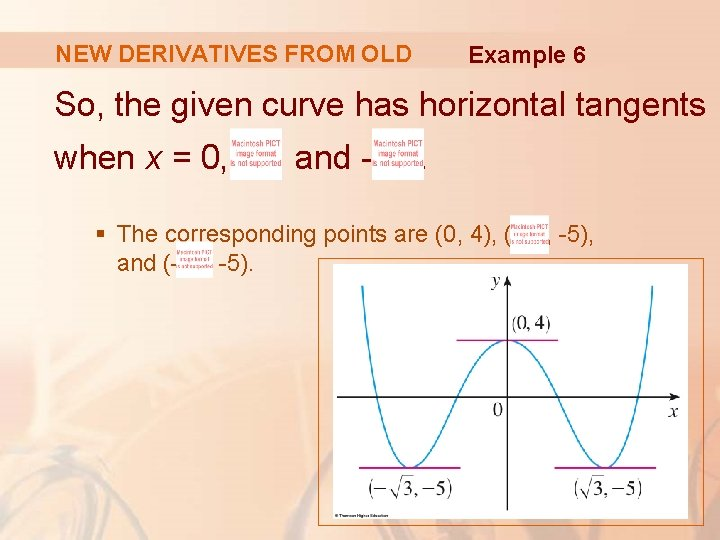NEW DERIVATIVES FROM OLD Example 6 So, the given curve has horizontal tangents when x = 0, , and - . § The corresponding points are (0, 4), ( and (- , -5),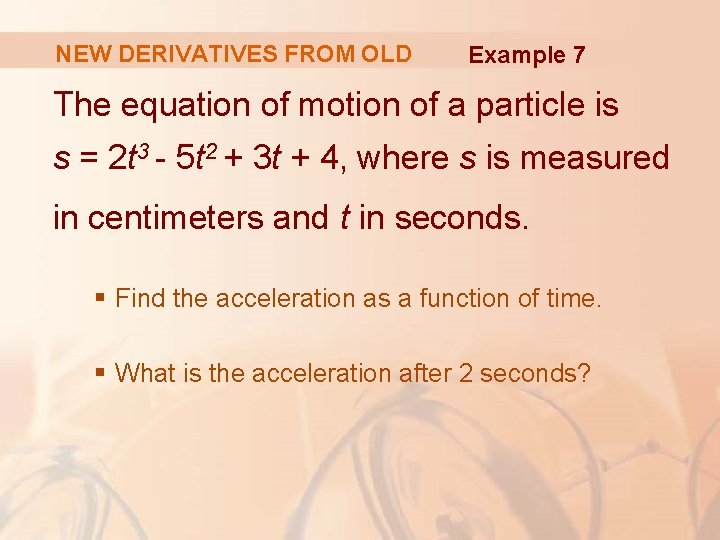NEW DERIVATIVES FROM OLD Example 7 The equation of motion of a particle is s = 2 t 3 - 5 t 2 + 3 t + 4, where s is measured in centimeters and t in seconds. § Find the acceleration as a function of time. § What is the acceleration after 2 seconds?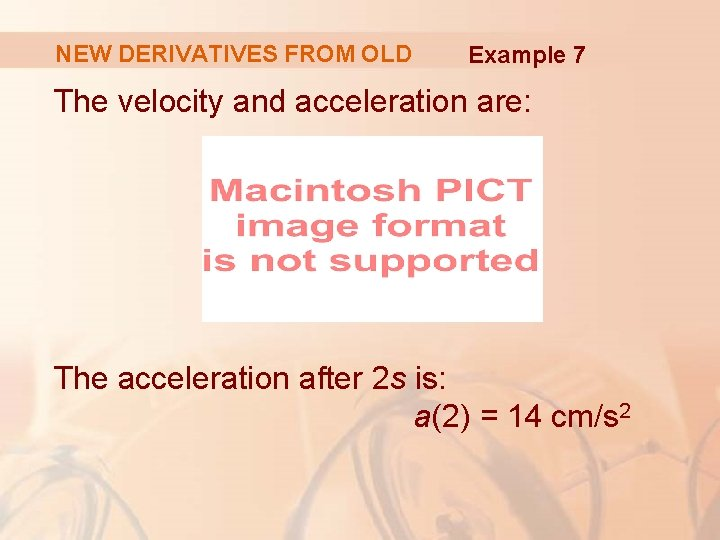NEW DERIVATIVES FROM OLD Example 7 The velocity and acceleration are: The acceleration after 2 s is: a(2) = 14 cm/s 2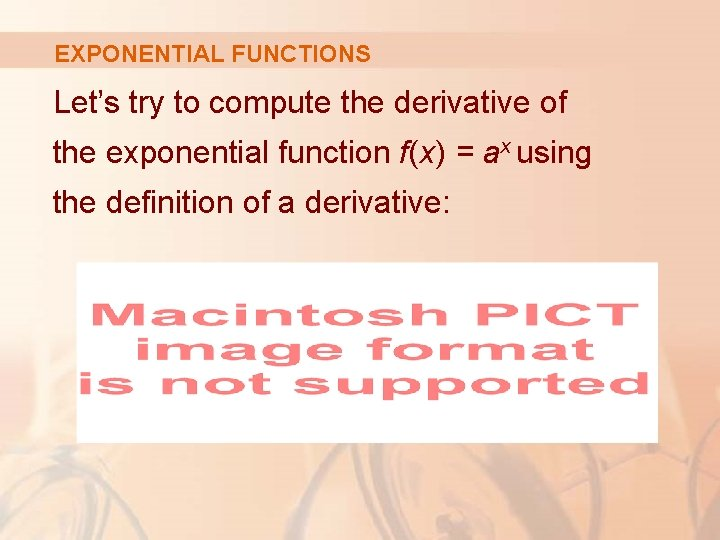EXPONENTIAL FUNCTIONS Let’s try to compute the derivative of the exponential function f(x) = ax using the definition of a derivative: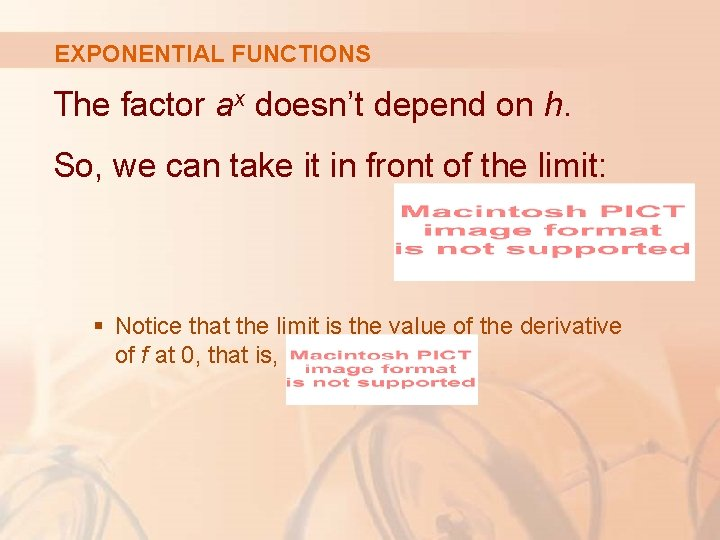EXPONENTIAL FUNCTIONS The factor ax doesn’t depend on h. So, we can take it in front of the limit: § Notice that the limit is the value of the derivative of f at 0, that is,EXPONENTIAL FUNCTIONS Equation 4 Thus, we have shown that, if the exponential function f(x) = ax is differentiable at 0, then it is differentiable everywhere and f’(x) = f’(0)ax § The equation states that the rate of change of any exponential function is proportional to the function itself. § The slope is proportional to the height.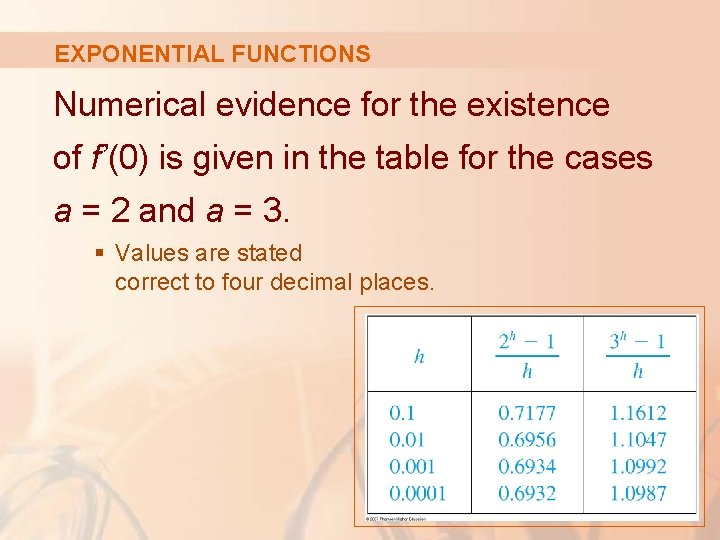EXPONENTIAL FUNCTIONS Numerical evidence for the existence of f’(0) is given in the table for the cases a = 2 and a = 3. § Values are stated correct to four decimal places.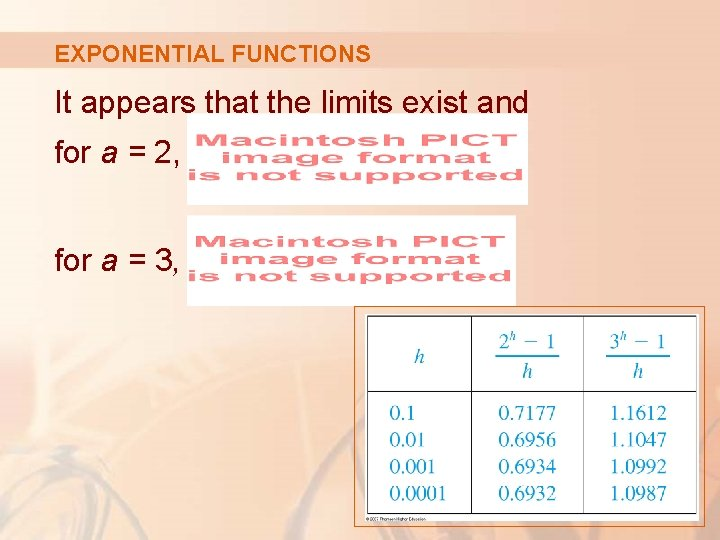EXPONENTIAL FUNCTIONS It appears that the limits exist and for a = 2, for a = 3,EXPONENTIAL FUNCTIONS In fact, it can be proved that these limits exist and, correct to six decimal places, the values are:EXPONENTIAL FUNCTIONS Thus, from Equation 4, we have: Equation 5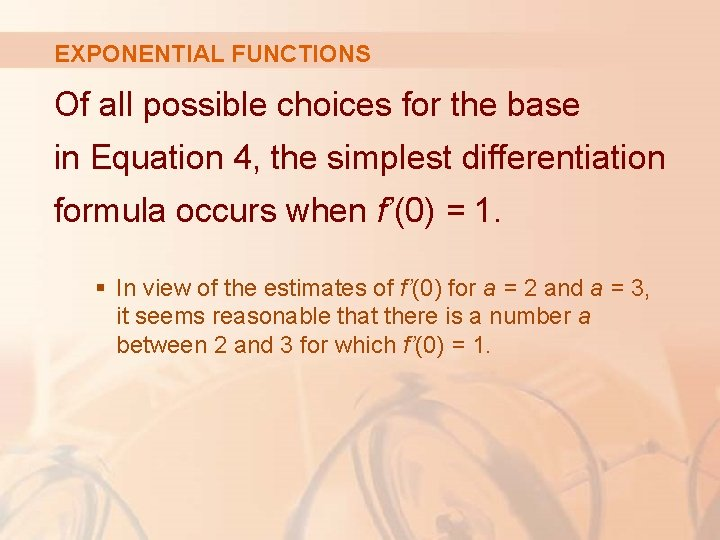EXPONENTIAL FUNCTIONS Of all possible choices for the base in Equation 4, the simplest differentiation formula occurs when f’(0) = 1. § In view of the estimates of f’(0) for a = 2 and a = 3, it seems reasonable that there is a number a between 2 and 3 for which f’(0) = 1.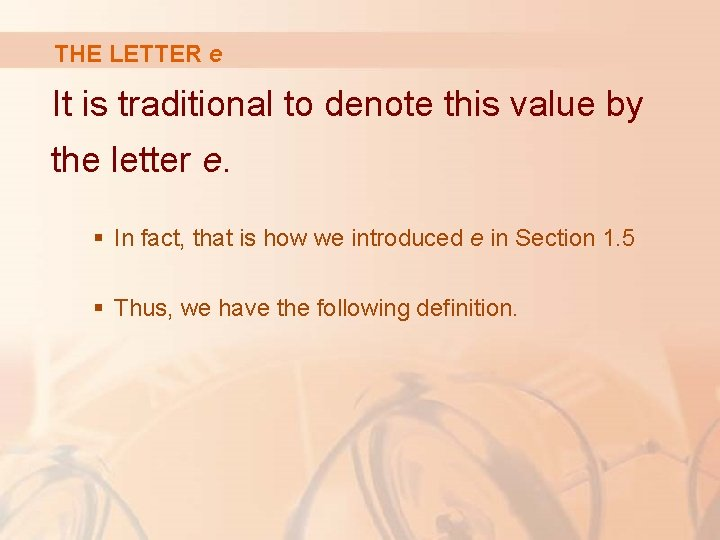THE LETTER e It is traditional to denote this value by the letter e. § In fact, that is how we introduced e in Section 1. 5 § Thus, we have the following definition.DEFINITION OF THE NUMBER e e is the number such that:EXPONENTIAL FUNCTIONS Geometrically, this means that, of all the possible exponential functions y = ax, the function f(x) = ex is the one whose tangent line at (0, 1) has a slope f’(0) that is exactly 1.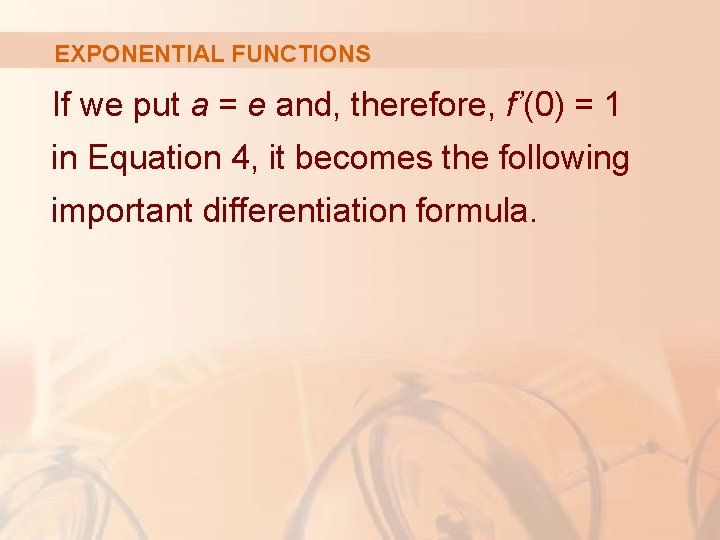EXPONENTIAL FUNCTIONS If we put a = e and, therefore, f’(0) = 1 in Equation 4, it becomes the following important differentiation formula.DERIV. OF NATURAL EXPONENTIAL FUNCTION The derivative of the natural exponential function is:EXPONENTIAL FUNCTIONS Thus, the exponential function f(x) = ex has the property that it is its own derivative. § The geometrical significance of this fact is that the slope of a tangent line to the curve y = ex is equal to the coordinate of the point.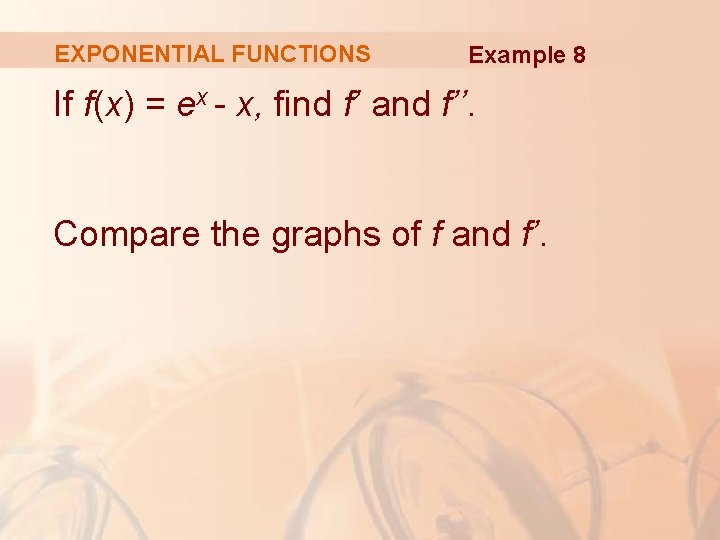EXPONENTIAL FUNCTIONS Example 8 If f(x) = ex - x, find f’ and f’’. Compare the graphs of f and f’.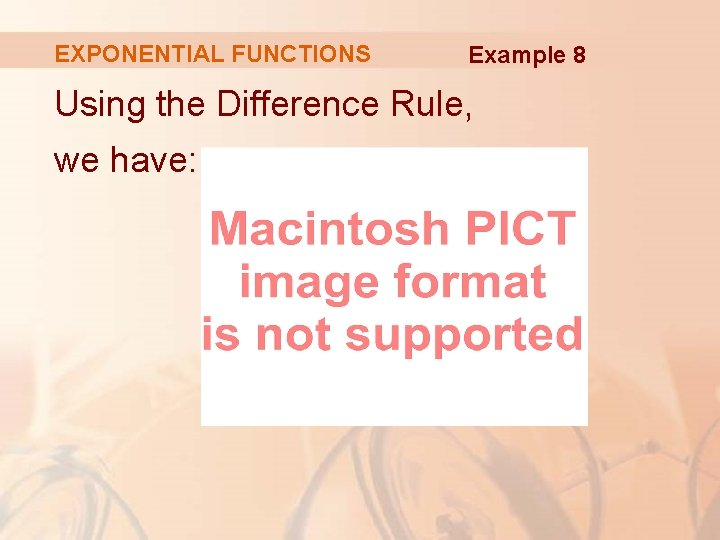EXPONENTIAL FUNCTIONS Example 8 Using the Difference Rule, we have: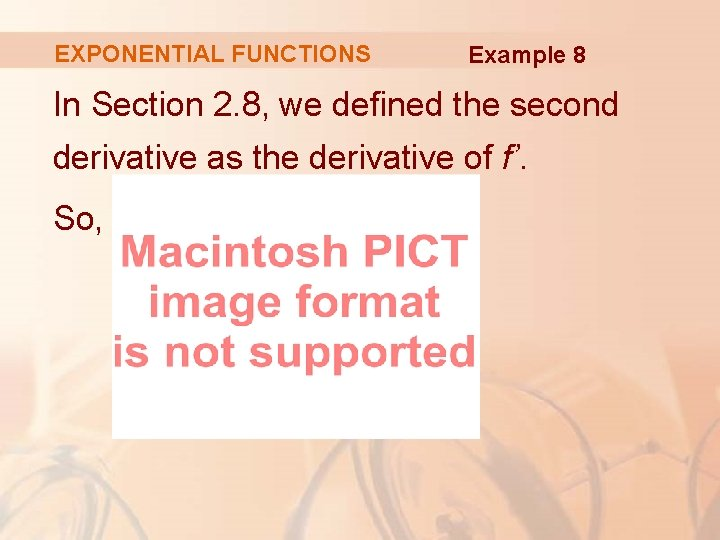EXPONENTIAL FUNCTIONS Example 8 In Section 2. 8, we defined the second derivative as the derivative of f’. So,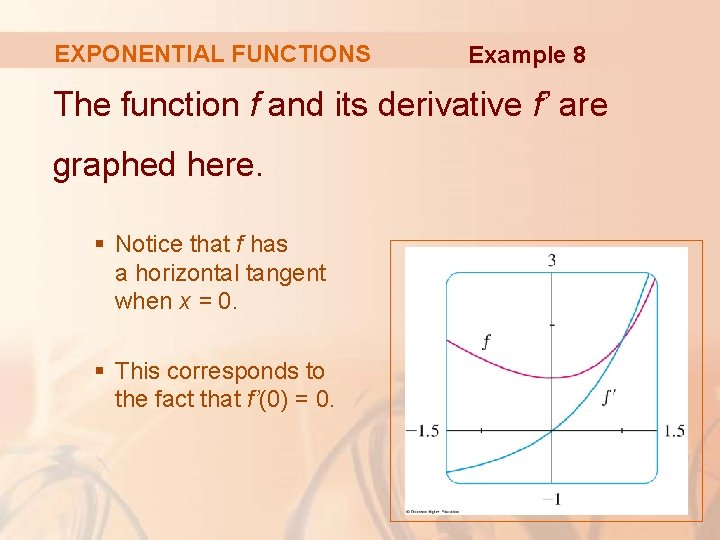EXPONENTIAL FUNCTIONS Example 8 The function f and its derivative f’ are graphed here. § Notice that f has a horizontal tangent when x = 0. § This corresponds to the fact that f’(0) = 0.EXPONENTIAL FUNCTIONS Example 8 Notice also that, for x > 0, f’(x) is positive and f is increasing. When x < 0, f’(x) is negative and f is decreasing.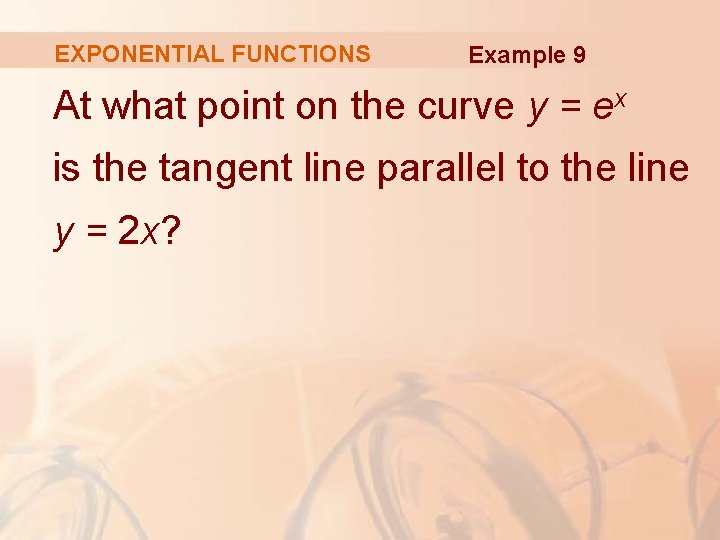EXPONENTIAL FUNCTIONS Example 9 At what point on the curve y = ex is the tangent line parallel to the line y = 2 x?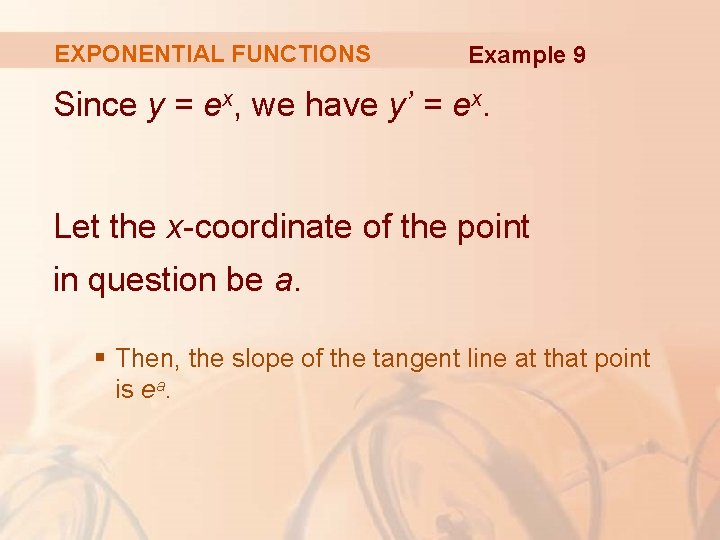EXPONENTIAL FUNCTIONS Example 9 Since y = ex, we have y’ = ex. Let the x-coordinate of the point in question be a. § Then, the slope of the tangent line at that point is ea.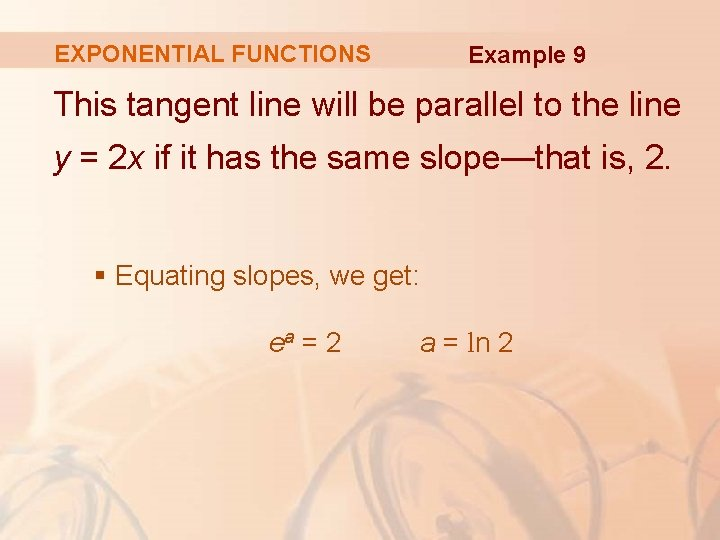EXPONENTIAL FUNCTIONS Example 9 This tangent line will be parallel to the line y = 2 x if it has the same slope—that is, 2. § Equating slopes, we get: ea = 2 a = ln 2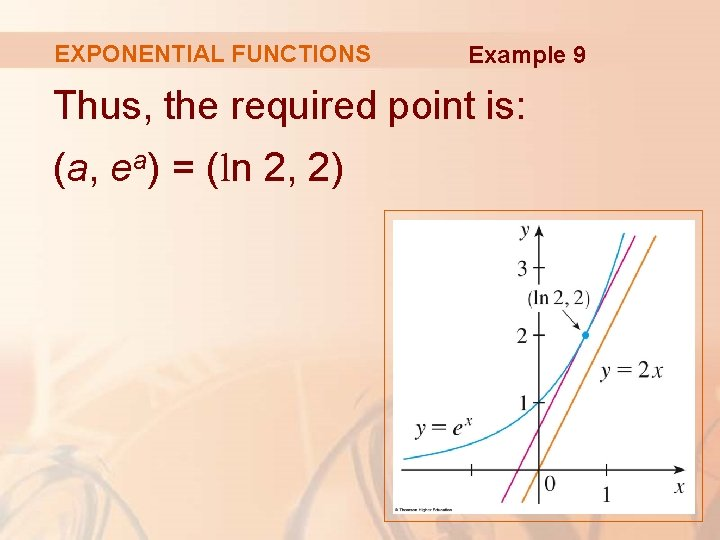EXPONENTIAL FUNCTIONS Example 9 Thus, the required point is: (a, ea) = (ln 2, 2)